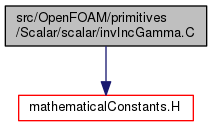The OpenFOAM Foundation
invIncGamma.C File Reference

Function to calculate the inverse of the normalized incomplete gamma function. More...

Include dependency graph for invIncGamma.C:Go to the source code of this file.

## Namespaces

Foam
Namespace for OpenFOAM.

## Functions

static scalar minimaxs (const scalar P)

static scalar Sn (const scalar a, const scalar x)

## Detailed Description

Function to calculate the inverse of the normalized incomplete gamma function.

Original source file invIncGamma.C

The algorithm is described in detain in reference:

```    DiDonato, A. R., & Morris Jr, A. H. (1986).
Computation of the incomplete gamma function ratios and their inverse.
ACM Transactions on Mathematical Software (TOMS), 12(4), 377-393.
```

All equation numbers in the following code refer to the above text and the algorithm in the function 'invIncGamma' is described in section 4.

Definition in file invIncGamma.C.